# 什么是SVG

• 位图：放大会失真图像边缘有锯齿；是由像素点组成；前端的 `Canvas` 就是位图效果。
• 矢量图：放大不会失真；使用 `XML` 描述图形。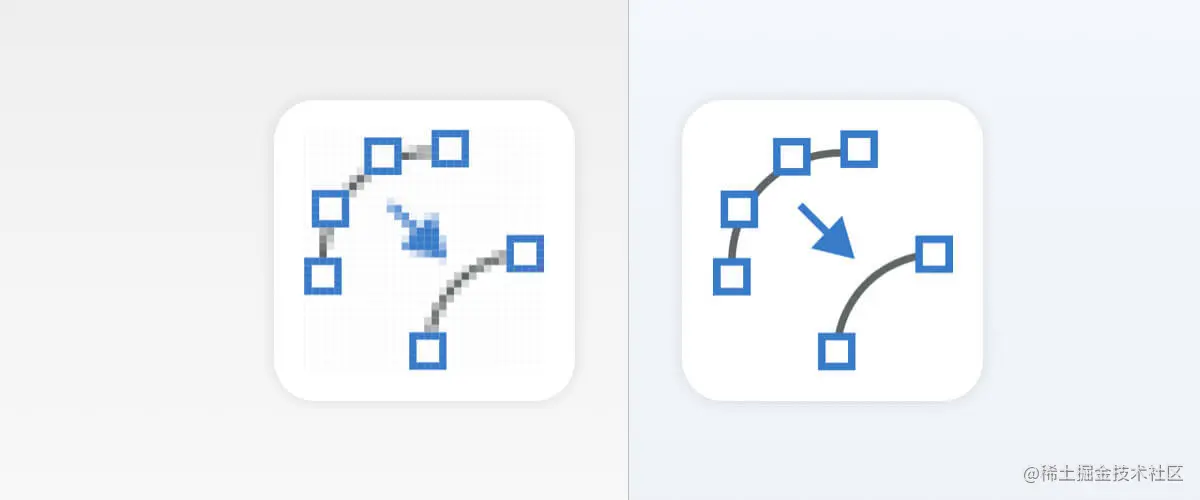# SVG的使用方式

## 使用方法

`SVG` 的使用方式有很多种，我最推荐直接在 `HTML` 中直接使用，也就是直接当 `HTML` 标签使用。

1. 在浏览器直接打开
2. 内嵌到 `HTML` 中（推荐⭐⭐⭐）
3. `CSS` 背景图（推荐⭐）
4. 使用 `img` 标签引入（推荐⭐）
5. 使用 `iframe` 标签引入（不推荐❌）
6. 使用 `embed` 标签引入（不推荐❌）
7. 使用 `object` 标签引入（不推荐❌）

## SVG默认宽高

`HTML` 中使用 `SVG` ，直接用 `<svg>` 标签即可。

``````<svg></svg>
``````

# 基础图形

`HTML` 的元素大多数默认都是矩形，`SVG` 在形状上更加丰富。

## 矩形 rect

• `x`: 左上角x轴坐标
• `y`: 左上角y轴坐标
• `width`: 宽度
• `height`: 高度
• `rx`: 圆角，x轴的半径
• `ry`: 圆角，y轴的半径

### 基础款（只设置宽高）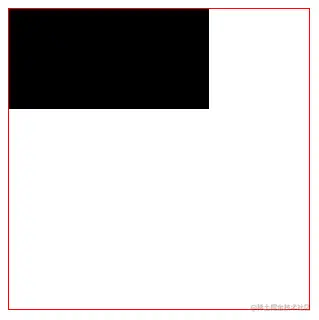``````<svg width="300" height="300" style="border: 1px solid red;">
<rect width="200" height="100"></rect>
</svg>
``````

### 设置矩形位置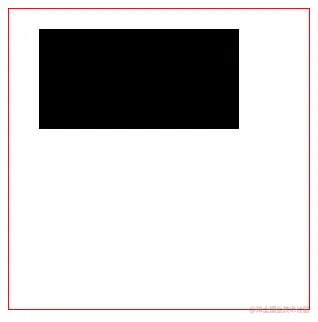``````<svg width="300" height="300" style="border: 1px solid red;">
<rect
x="30"
y="20"
width="200"
height="100"
>
</rect>
</svg>
``````

### 圆角矩形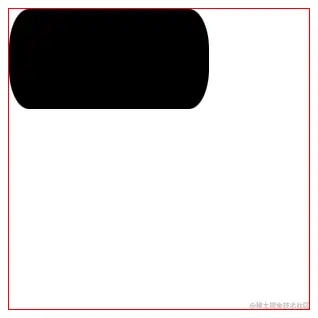``````<svg width="300" height="300" style="border: 1px solid red;">
<rect
width="200"
height="100"
rx="20"
ry="40"
>
</rect>
</svg>
``````

`rx` 是设置x轴的半径，`ry` 设置y轴的半径。

`rx` 的最大值是矩形宽度的一半，`ry` 的最大值是矩形高度的一半。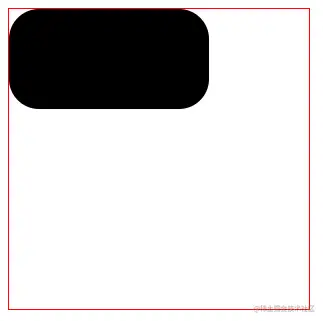``````<svg width="300" height="300" style="border: 1px solid red;">
<rect
width="200"
height="100"
rx="30"
>
</rect>
</svg>
``````

### rect版的圆形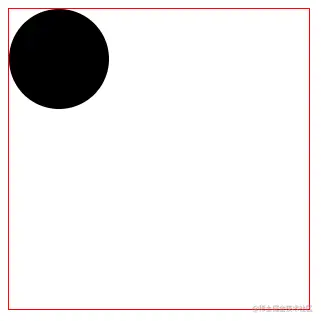``````<svg width="300" height="300" style="border: 1px solid red;">
<rect
width="100"
height="100"
rx="50"
>
</rect>
</svg>
``````

## 圆形 circle

• `cx`: 圆心在x轴的坐标
• `cy`: 圆心在y轴的坐标
• `r`: 半径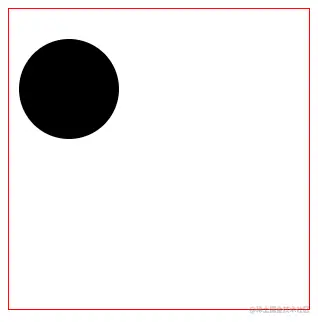``````<svg width="300" height="300" style="border: 1px solid red;">
<circle
cx="60"
cy="80"
r="50"
>
</circle>
</svg>
``````

## 椭圆 ellipse

• `cx`: 圆心在x轴的坐标
• `cy`: 圆心在y轴的坐标
• `rx`: x轴的半径
• `ry`: y轴的半径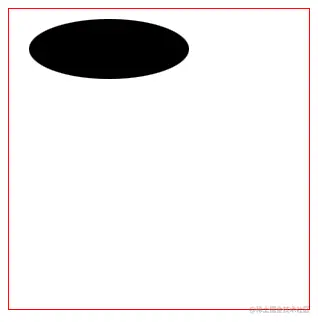``````<svg width="300" height="300" style="border: 1px solid red;">
<ellipse
cx="100"
cy="40"
rx="80"
ry="30"
>
</ellipse>
</svg>
``````

`<ellipse>``<circle>` 差不多，只是将半径拆成x轴和y轴的。

## 直线 line

• `x1`: 起始点x坐标
• `y1`: 起始点y坐标
• `x2`: 结束点x坐标
• `y2`: 结束点y坐标
• `stroke`: 描边颜色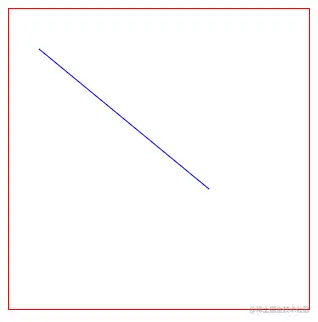``````<svg width="300" height="300" style="border: 1px solid red;">
<line
x1="30"
y1="40"
x2="200"
y2="180"
stroke="blue"
>
</line>
</svg>
``````

## 折线 polyline

• `points`: 点集
• `stroke`: 描边颜色
• `fill`: 填充颜色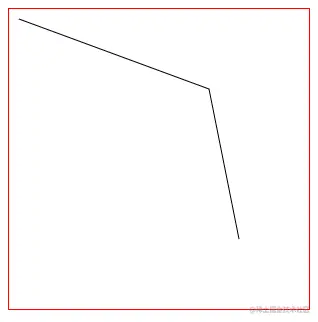``````<svg width="300" height="300" style="border: 1px solid red;">
<polyline
points="10 10, 200 80, 230 230"
stroke="#000"
fill="none"
>
</polyline>
</svg>
``````

`points` 接受的值是一串点集，点集是两两一组表示一个坐标。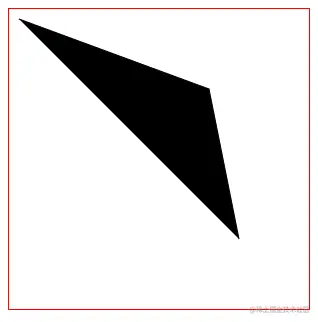``````<svg width="300" height="300" style="border: 1px solid red;">
<polyline
points="10 10, 200 80, 230 230"
stroke="#000"
>
</polyline>
</svg>
``````

`fill` 设置成 `none` 后，必须设置 `stroke` 为一个有颜色的值，不然不会有渲染效果。

## 多边形 polygon

• `points`: 点集
• `stroke`: 描边颜色
• `fill`: 填充颜色

`<polygon>` 会自动闭合（自动将起始点和结束点链接起来）。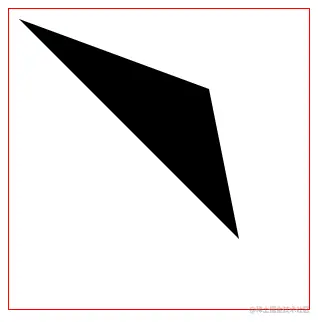``````<svg width="300" height="300" style="border: 1px solid red;">
<polygon points="10 10, 200 80, 230 230"></polygon>
</svg>
``````

## 直线路径 path

`d` 属性又包括以下主要的关键字（注意大小写！）：

• M: 起始点坐标，`moveto` 的意思。每个路径都必须以 `M` 开始。`M` 传入 `x``y` 坐标，用逗号或者空格隔开。
• `L`: 轮廓坐标，`lineto` 的意思。`L` 是跟在 `M` 后面的。它也是可以传入一个或多个坐标。大写的 `L` 是一个绝对位置
• l: 这是小写 `L`，和 `L` 的作用差不多，但 `l` 是一个相对位置
• `H`: 和上一个点的Y坐标相等，是 `horizontal lineto` 的意思。它是一个绝对位置
• `h`: 和 `H` 差不多，但 `h` 使用的是相对定位
• `V`: 和上一个点的X坐标相等，是`vertical lineto` 的意思。它是一个绝对位置
• `v`: 这是一个小写的 `v` ，和大写 `V` 的差不多，但小写 `v` 是一个相对定位。
• `Z`: 关闭当前路径，`closepath` 的意思。它会绘制一条直线回到当前子路径的起点。

### 基础版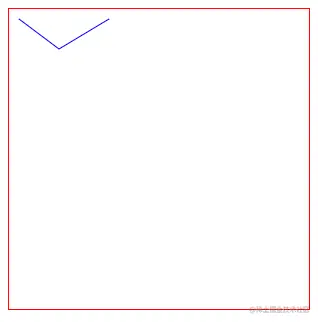``````<svg width="300" height="300" style="border: 1px solid red;">
<path
d="M 10 10 L 50 40 L 100 10"
stroke="blue"
fill="none"
>
</path>
</svg>
``````

### 简写``````<svg width="300" height="300" style="border: 1px solid red;">
<path
d="M 10 10 50 40 100 10"
stroke="blue"
fill="none"
>
</path>
</svg>
``````

### 闭合路径

`d` 的数据集里，使用 `Z` 可以闭合路径。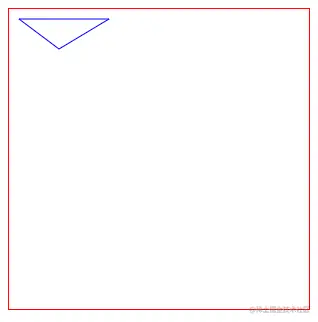``````<svg width="300" height="300" style="border: 1px solid red;">
<path
d="M 10 10 L 50 40 L 100 10 Z"
stroke="blue"
fill="none"
>
</path>
</svg>
``````

### 轮廓坐标相对位置 l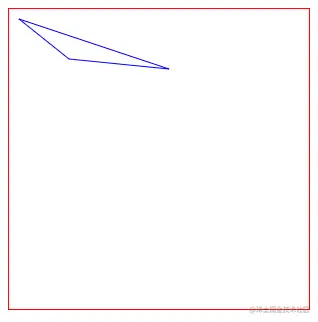``````<svg width="300" height="300" style="border: 1px solid red;">
<path
d="M 10 10 l 50 40 l 100 10 Z"
stroke="blue"
fill="none"
>
</path>
</svg>
``````

`l` 里的参数会与前一个点的 `x``y` 进行相加，得到一个新的坐标。

### H 和 h

`H` 后面只需传入 `X坐标` 即可，它的 `Y坐标` 与前一个点相同。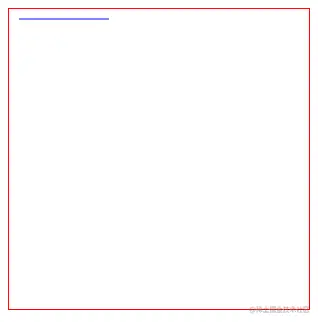``````<svg width="300" height="300" style="border: 1px solid red;">
<path
d="M 10 10 H 100"
stroke="blue"
fill="none"
>
</path>
</svg>
``````

`h``H` 的作用差不多，只不过传入的数据会和前一个点的 `X坐标` 相加，形成一个新的点，这就是相对位置。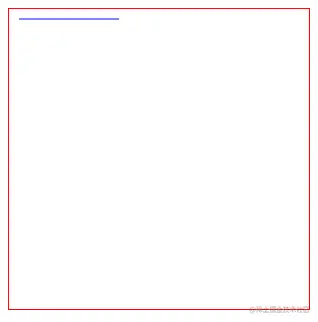``````<svg width="300" height="300" style="border: 1px solid red;">
<path
d="M 10 10 h 100"
stroke="blue"
fill="none"
>
</path>
</svg>
``````

### V 和 v

`V` 后面只需传入 `Y坐标` 即可，它的 `X坐标` 与前一个点相同。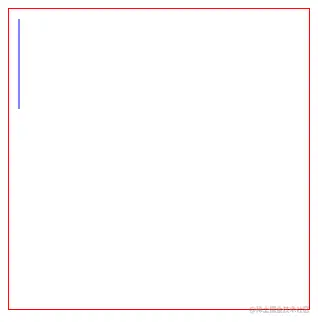``````<svg width="300" height="300" style="border: 1px solid red;">
<path
d="M 10 10 V 100"
stroke="blue"
fill="none"
>
</path>
</svg>
``````

`v``V` 的作用差不多，小写 `v` 是一个相对位置。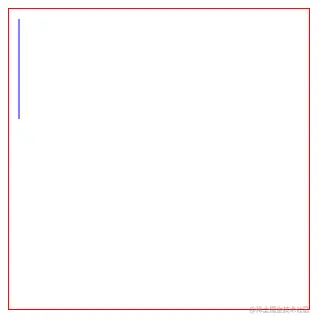``````<svg width="300" height="300" style="border: 1px solid red;">
<path
d="M 10 10 v 100"
stroke="blue"
fill="none"
>
</path>
</svg>
``````

## 曲线 - 椭圆弧路径 path

`SVG` 中，画曲线其实有很多种方法。我觉得最简单的是 椭圆弧曲线，其实还有 贝塞尔曲线三次贝塞尔曲线 等一系列复杂的曲线。但我觉得这对初学者来说可能一下子难以接受，所以我在 《Canvas 从入门到劝朋友放弃（图解版）》 里也没写。之后打算再写一篇贝塞尔曲线相关的文章骗点赞~

### 什么是椭圆弧？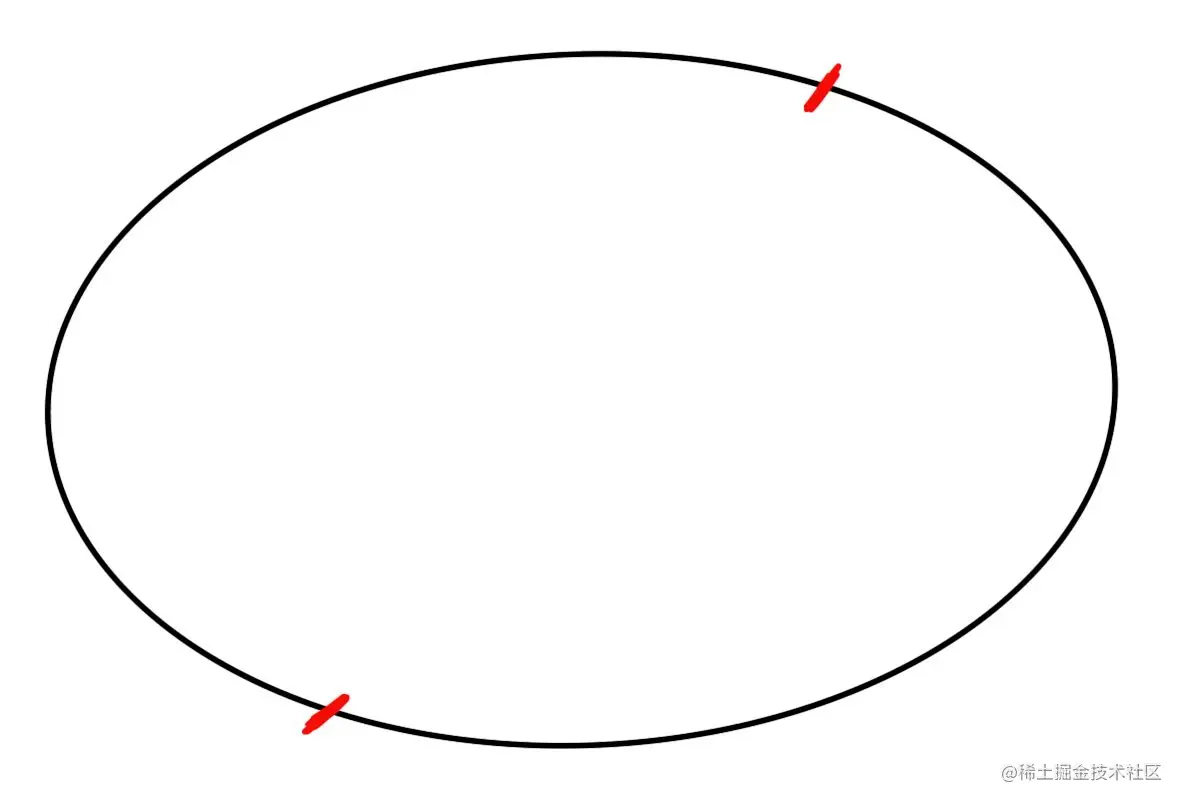### 椭圆弧公式

`SVG` 中可以使用 `path` 配合 `A属性` 绘制椭圆弧。

``````A(rx, ry, xr, laf, sf, x, y)
``````
• `rx`: 椭圆X轴半径
• `ry`: 椭圆Y轴半径
• `xr`: 椭圆旋转角度
• `laf`: 是否选择弧长较长的那一段。0: 短边（小于180度）; 1: 长边（大于等于180度）
• `sf`: 是否顺时针绘制。0: 逆时针; 1: 顺时针
• `x`: 终点X轴坐标
• `y`: 终点Y轴坐标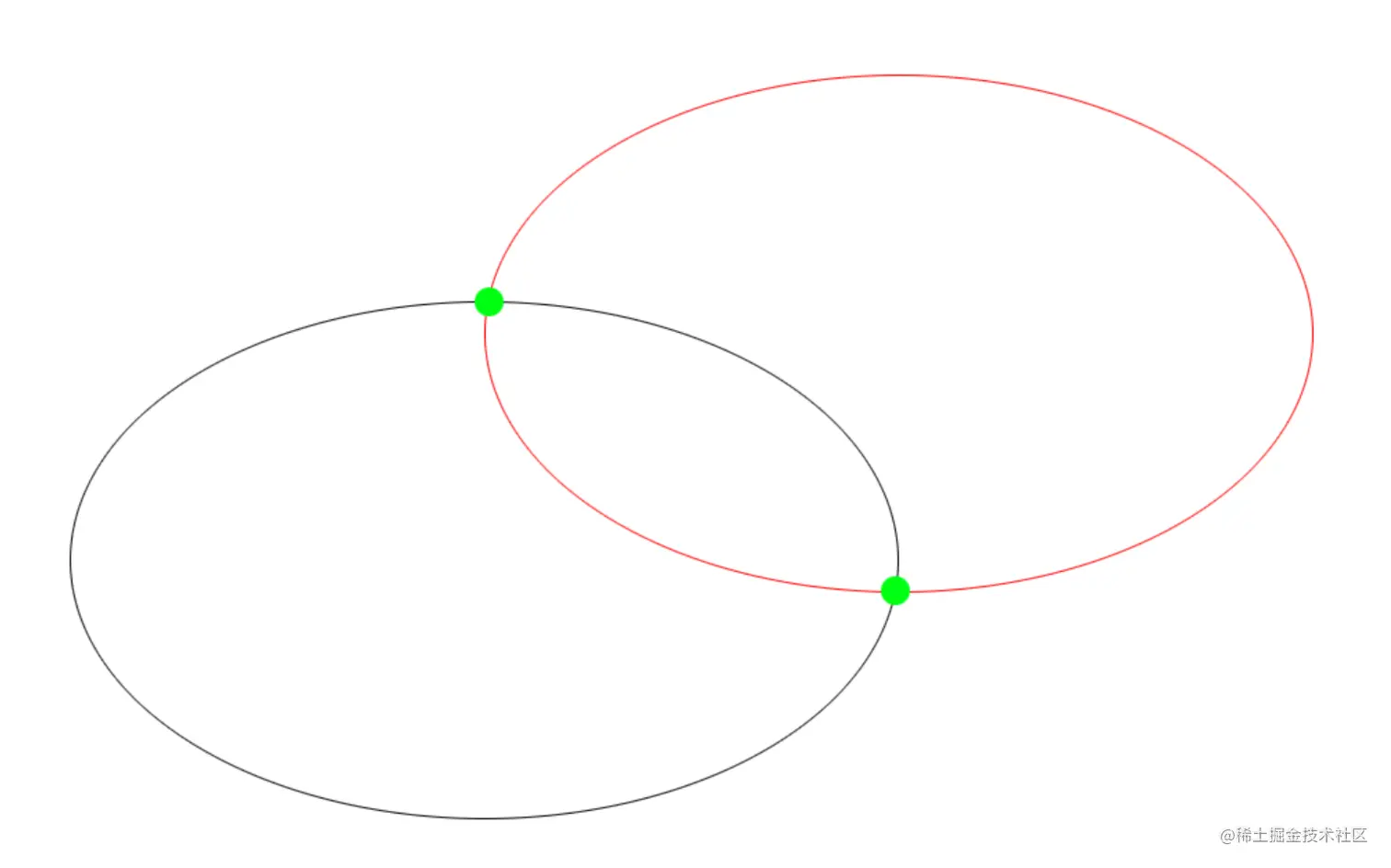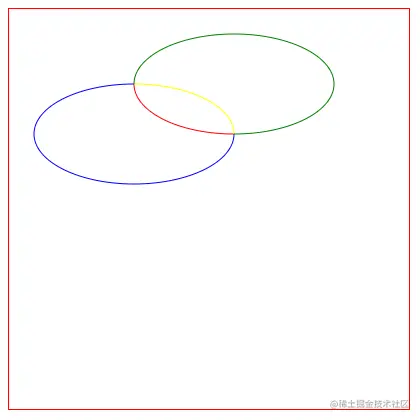``````<svg width="400" height="400" style="border: 1px solid red;">
<!-- 红 -->
<path
d="M 125 75 A 100 50 0 0 0 225 125"
stroke="red"
fill="none"
/>

<!-- 黄 -->
<path
d="M 125 75 A 100 50 0 0 1 225 125"
stroke="yellow"
fill="none"
/>

<!-- 蓝 -->
<path
d="M 125 75 A 100 50 0 1 0 225 125"
stroke="blue"
fill="none"
/>

<!-- 绿 -->
<path
d="M 125 75 A 100 50 0 1 1 225 125"
stroke="green"
fill="none"
/>
</svg>
``````

# 设置样式的方法

• 属性样式
• 内联样式
• 内部样式
• 外部样式

## 属性样式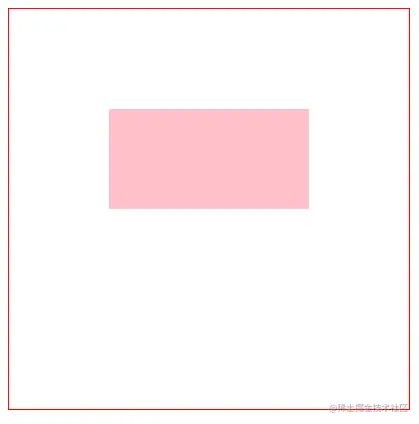``````<svg width="400" height="400" style="border: 1px solid red;">
<rect
x="100"
y="100"
width="200"
height="100"
fill="pink"
/>
</svg>
``````

## 内联样式

``````<svg width="400" height="400" style="border: 1px solid red;">
<rect
x="100"
y="100"
width="200"
height="100"
style="fill: pink;"
/>
</svg>
``````

## 内部样式

``````<style>
.rect {
fill: pink;
}
</style>

<svg width="400" height="400" style="border: 1px solid red;">
<rect
x="100"
y="100"
width="200"
height="100"
class="rect"
/>
</svg>
``````

# 常用样式设置

`SVG` 设置样式的属性和 `CSS` 稍微有点不同，但初学时不需要了解太深入，我们只需将常用的学会即可。

• 关键字: red、pink、blue 等
• 十六进制: 支持3位或6位，`#0f0``#00ff00`
• RGB 和 RGBA: 比如 `rgb(10, 20, 30)``rgba(10, 20, 30, 0.4)`
• HSL 和 HSLA

## 填充 fill

`fill` 默认是 `#000000` ，也就是黑色。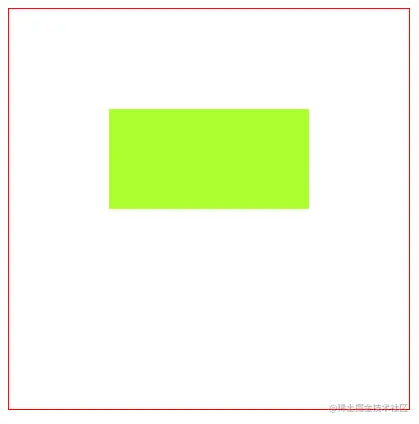``````<svg width="400" height="400" style="border: 1px solid red;">
<rect
x="100"
y="100"
width="200"
height="100"
fill="greenyellow"
/>
</svg>
``````

## 填充色的不透明度 fill-opacity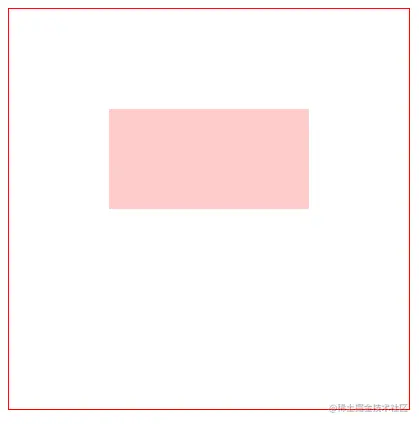``````<svg width="400" height="400" style="border: 1px solid red;">
<rect
x="100"
y="100"
width="200"
height="100"
fill="red"
fill-opacity="0.2"
/>
</svg>
``````

`fill` 属性中使用 `RGBA` 或者 `HSLA` 的方式你自己动手试试看~

## 描边颜色 stroke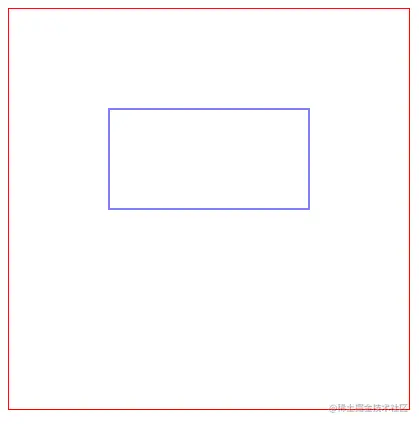``````<svg width="400" height="400" style="border: 1px solid red;">
<rect
x="100"
y="100"
width="200"
height="100"
fill="none"
stroke="blue"
/>
</svg>
``````

## 描边颜色的不透明度 stroke-opacity

`fill-opacity` 差不多，只不过 `stroke-opacity` 是设置描边的不透明度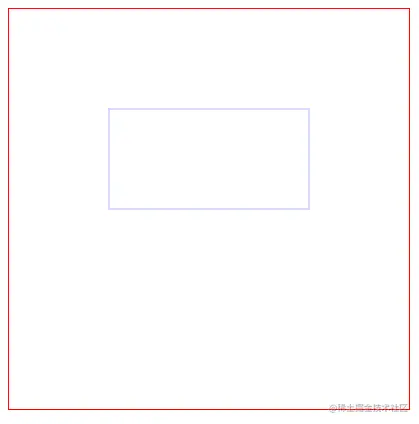``````<svg width="400" height="400" style="border: 1px solid red;">
<rect
x="100"
y="100"
width="200"
height="100"
fill="none"
stroke="blue"
stroke-opacity="0.3"
/>
</svg>
``````

## 描边宽度 stroke-width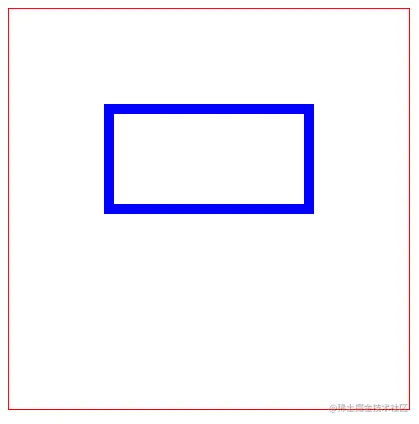``````<svg width="400" height="400" style="border: 1px solid red;">
<rect
x="100"
y="100"
width="200"
height="100"
fill="none"
stroke="blue"
stroke-width="10"
/>
</svg>
``````

## 虚线描边 stroke-dasharray

`stroke-dasharray` 接收一串数字，这串数字可以用来代表 线的长度和空隙的长度，数字之间用逗号或者空格分隔。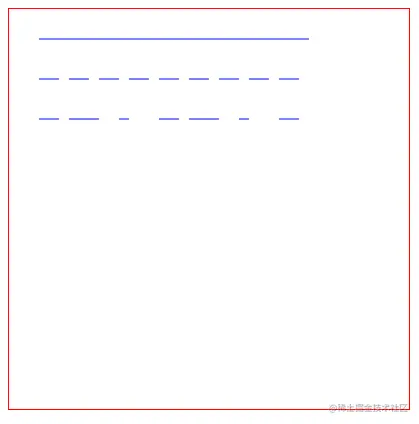``````<svg width="400" height="400" style="border: 1px solid red;">
<line
x1="30"
y1="30"
x2="300"
y2="30"
stroke="blue"
/>

<line
x1="30"
y1="70"
x2="300"
y2="70"
stroke="blue"
stroke-dasharray="20 10"
/>

<line
x1="30"
y1="110"
x2="300"
y2="110"
stroke="blue"
stroke-dasharray="20 10 30"
/>
</svg>
``````

## 虚线偏移量 stroke-dashoffset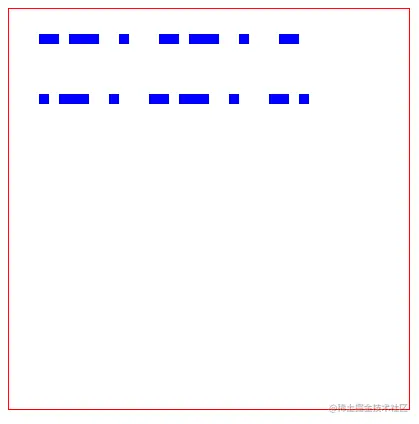``````<svg width="400" height="400" style="border: 1px solid red;">
<line
x1="30"
y1="30"
x2="300"
y2="30"
stroke="blue"
stroke-width="10"
stroke-dasharray="20 10 30"
/>

<line
x1="30"
y1="90"
x2="300"
y2="90"
stroke="blue"
stroke-width="10"
stroke-dasharray="20 10 30"
stroke-dashoffset="10"
/>
</svg>
``````

## 线帽 stroke-linecap

• `butt`: 平头（默认值）
• `round`: 圆头
• `square`: 方头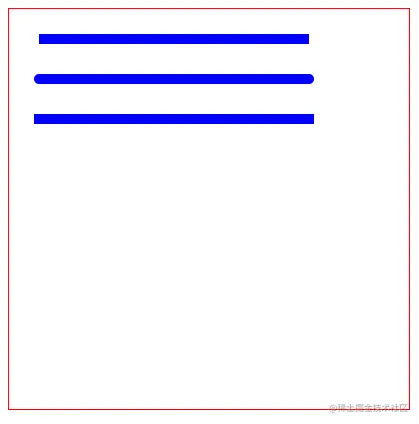``````<svg width="400" height="400" style="border: 1px solid red;">
<!-- 平头 -->
<line
x1="30"
y1="30"
x2="300"
y2="30"
stroke="blue"
stroke-width="10"
stroke-linecap="butt"
/>

<!-- 圆头 -->
<line
x1="30"
y1="70"
x2="300"
y2="70"
stroke="blue"
stroke-width="10"
stroke-linecap="round"
/>

<!-- 方头 -->
<line
x1="30"
y1="110"
x2="300"
y2="110"
stroke="blue"
stroke-width="10"
stroke-linecap="square"
/>
</svg>
``````

## 拐角 stroke-linejoin

• `miter`: 尖角（默认）
• `round`: 圆角
• `bevel`: 平角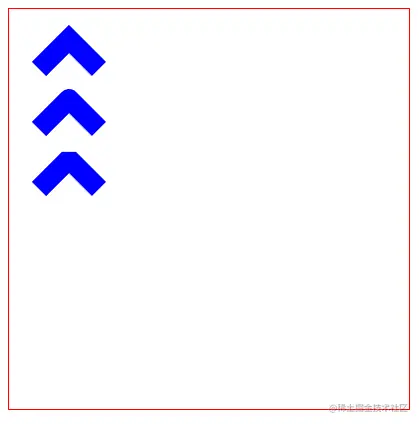``````<svg width="400" height="400" style="border: 1px solid red;">
<!-- 尖角 -->
<polyline
points="30 60, 60 30, 90 60"
fill="none"
stroke="blue"
stroke-width="20"
stroke-linejoin="miter"
/>

<!-- 圆角 -->
<polyline
points="30 120, 60 90, 90 120"
fill="none"
stroke="blue"
stroke-width="20"
stroke-linejoin="round"
/>

<!-- 平角 -->
<polyline
points="30 180, 60 150, 90 180"
fill="none"
stroke="blue"
stroke-width="20"
stroke-linejoin="bevel"
/>
</svg>
``````

## 消除锯齿 shape-rendering

``````shape-rendering: crispEdges;
``````

# 文本元素 text

`SVG` 可以使用 `<text>` 标签渲染文本。文本是有 “基线” 概念的，这个概念和 `CSS` 的一样。这里推荐 AndyHu 的文章，可以快速搞懂基线。《彻底搞懂vertical-align 底线、基线、中线的含义》

## 基础版

`Canvas` 一样，`SVG` 的文本对齐方式是以第一个字基线的左下角为基准。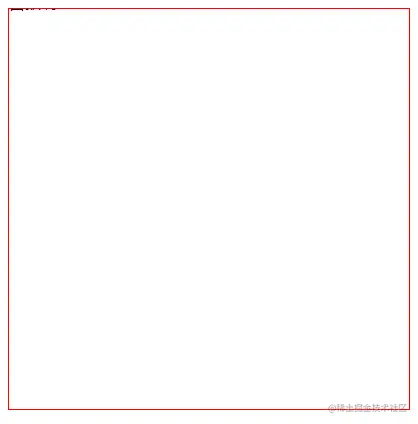``````<svg width="400" height="400" style="border: 1px solid red;">
<text>雷猴啊</text>
</svg>
``````

`SVG` 如果没设置字号，它会跟随父元素的字号，一直往上跟跟跟上去。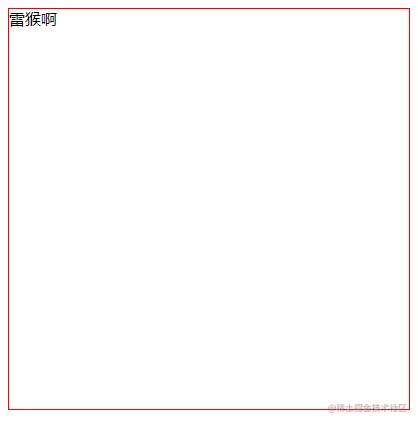``````<svg width="400" height="400" style="border: 1px solid red;">
<text y="16">雷猴啊</text>
</svg>
``````

## 设置字号 font-size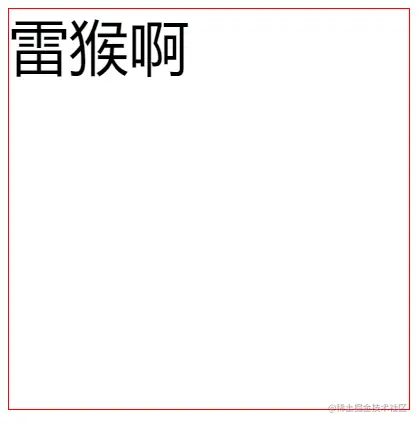``````<svg width="400" height="400" style="border: 1px solid red;">
<text
y="60"
font-size="60"
>
雷猴啊
</text>
</svg>
``````

## 粗体 font-weight

• `normal`: 默认（非粗体）
• `bold`: 粗体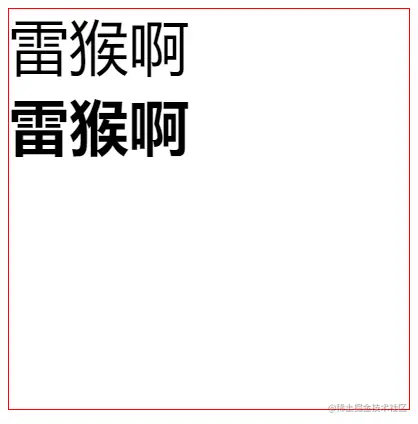``````<svg width="400" height="400" style="border: 1px solid red;">
<!-- 默认 -->
<text
y="60"
font-size="60"
font-weight="normal"
>
雷猴啊
</text>

<!-- 粗体 -->
<text
y="140"
font-size="60"
font-weight="bold"
>
雷猴啊
</text>
</svg>
``````

## 装饰线 text-decoration

`CSS` 一样，可以使用 `text-decoration` 设置装饰线

• `none`：默认
• `underline`: 下划线
• `overline`: 上划线
• `line-through`: 删除线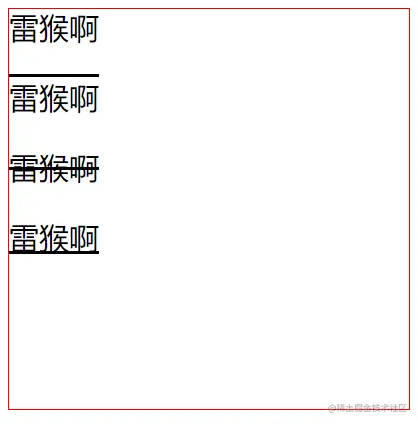``````<svg width="400" height="400" style="border: 1px solid red;">
<!-- 默认 -->
<text
y="30"
font-size="30"
text-decoration="none"
>
雷猴啊
</text>

<!-- 上划线 -->
<text
y="100"
font-size="30"
text-decoration="overline"
>
雷猴啊
</text>

<!-- 删除线 -->
<text
y="170"
font-size="30"
text-decoration="line-through"
>
雷猴啊
</text>

<!-- 下划线 -->
<text
y="240"
font-size="30"
text-decoration="underline"
>
雷猴啊
</text>
</svg>
``````

## 水平对齐方式 text-anchor

• `start`: 左对齐
• `middle`: 居中对齐
• `end`: 右对齐

## 多行文本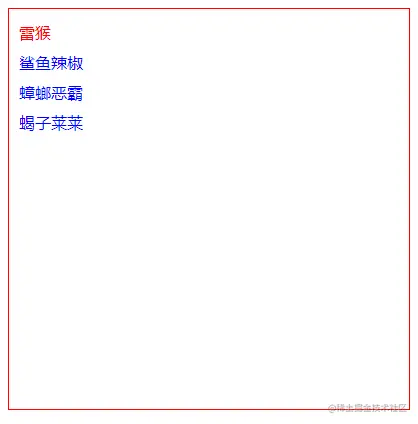``````<svg width="400" height="400" style="border: 1px solid red;">
<text fill="blue">
<tspan x="10" y="30" fill="red">雷猴</tspan>
<tspan x="10" y="60">鲨鱼辣椒</tspan>
<tspan x="10" y="90">蟑螂恶霸</tspan>
<tspan x="10" y="120">蝎子莱莱</tspan>
</text>
</svg>
``````

`<tspan>` 要放在 `<text>` 里，而且会继承 `<text>` 设置的样式。

## 水平对齐方式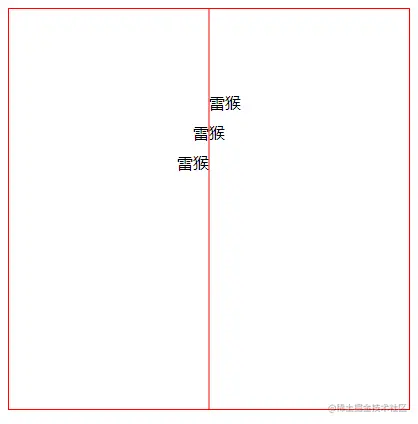``````<svg width="400" height="400" style="border: 1px solid red;">
<!-- 参考线 -->
<path d="M 200 0 200 400" stroke="red"></path>

<!-- 左对齐 -->
<text
x="200"
y="100"
text-anchor="start"
>
雷猴
</text>

<!-- 居中对齐 -->
<text
x="200"
y="130"
text-anchor="middle"
>
雷猴
</text>

<!-- 右对齐 -->
<text
x="200"
y="160"
text-anchor="end"
>
雷猴
</text>
</svg>
``````

## 垂直对齐方式 dominant-baseline

• `auto`: 默认的对齐方式，保持与父元素相同的配置。
• `text-after-edge`: 在基线上方
• `middle`: 居中基线
• `text-before-edge`: 在基线下方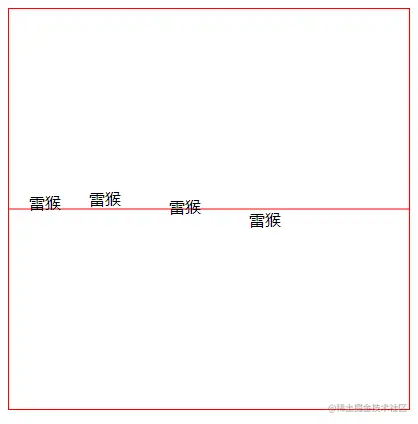``````<svg width="400" height="400" style="border: 1px solid red;">
<!-- 参考线 -->
<path d="M 0 200 400 200" stroke="red"></path>

<!-- 默认 -->
<text
x="20"
y="200"
dominant-baseline="auto"
>
雷猴
</text>

<!-- 在基线上方 -->
<text
x="80"
y="200"
dominant-baseline="text-after-edge"
>
雷猴
</text>

<!-- 居中基线 -->
<text
x="160"
y="200"
dominant-baseline="middle"
>
雷猴
</text>

<!-- 在基线下方 -->
<text
x="240"
y="200"
dominant-baseline="text-before-edge"
>
雷猴
</text>
</svg>
``````

## 纵向文字 writing-mode

`writing-mode` 设置成 `tb` 就可以让文字纵向排列。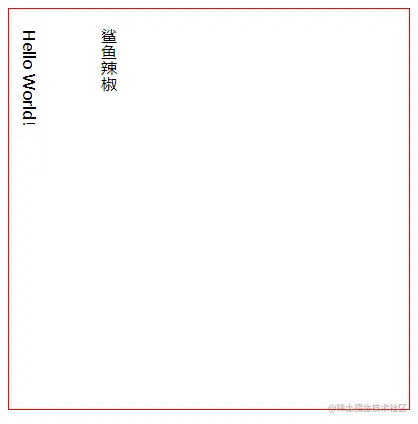``````<svg width="400" height="400" style="border: 1px solid red;">
<text x="20" y="20" writing-mode="tb">Hello World!</text>
<text x="100" y="20" writing-mode="tb">鲨鱼辣椒</text>
</svg>
``````

# 超链接

`HTML` 一样，超链接可以使用 `<a>` 标签实现。

`SVG` 里，链接要放在 `xlink:href` 属性里。``````<svg width="400" height="400" style="border: 1px solid red;">
<text x="20" y="20">也学学Canvas吧</text>
</a>
</svg>
``````

`<a>` 标签里除了可以包裹文本外，还可以包裹各种图形和图片等元素。

# 图片 image

`SVG` 中可以使用 `<image>` 标签加载图片，包括位图。

`<image>` 是使用 `xlink:href` 属性获取图片的``````<svg width="400" height="400" style="border: 1px solid red;">
</svg>
``````